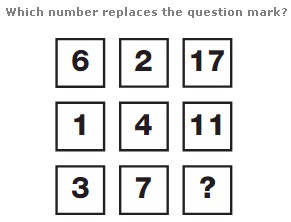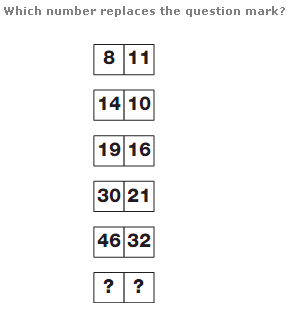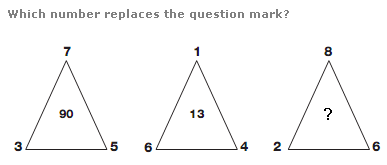# Puzzles - Number puzzles

### Exercise :: Number puzzlesAnswer : 21 Explanation : In each row, add the left and central numbers together, double it and add 1 to give the right hand value.Answer : 73, 48 Explanation : Starting at the top, add the left and right hand numbers together and subtract 5 to give the lower left number. Add 2 to the left hand number to give the lower right hand number.Answer : 80 Explanation : In each triangle, the central value equals the product of the 3 outer numbers subtracting the sum of the 3 outer numbers.